# 【机器学习基础】第四课：统计学知识之假设检验

## t检验，方差分析，卡方检验，秩检验

Posted by x-jeff on January 30, 2019

【机器学习基础】系列博客为参考周志华老师的《机器学习》一书，自己所做的读书笔记。

# 2.假设检验

1. $\mu=\mu_0$：即检验假设，常称无效假设或零（原）假设，用$H_0$表示。
2. $\mu\neq \mu_0$（或$\mu>\mu_0$，$\mu<\mu_0$等）：即备择假设，常称对立假设，用$H_1$或$H_A$表示。

• 检验假设针对的是总体，而不是样本。
• $H_0$为无效假设，其假定通常是：某两个（或多个）总体参数相等，或两个总体参数之差为0，或某资料服从某一特定分布（如正态分布，泊松分布）或某些情况无效等。
• 不拒绝$H_0$不等于接受$H_0$。下结论时，对$H_0$只能说拒绝$H_0$或不拒绝$H_0$，而对$H_1$只能说接受$H_1$，除此之外的其他说法均不妥当。

1. 参数检验（parametric test
2. 非参数检验（nonparametric test

## 2.1.参数检验

⚠️使用t检验和F检验的两个前提条件：1）正态分布总体；2）方差齐性。

### 2.1.1.t检验，F检验前提条件检验

👉正态性检验：

1. 图示法
• 概率图（probability-probability plot,P-P plot
• 分位数图（quantile-quantile plot,Q-Q plot
2. 计算法
• 对偏度（skewness）和峰度（kurtosis）各用一个指标来评定，其中以矩法（method of moment），又称动差法，效率最高。
• 仅用一个指标来综合评定，其中以$W$检验法和$W’$检验法效率最高，适用于样本含量少于100的资料；$D$检验法效率也高，适用于样本含量$n$为10～2000的资料。

👉方差齐性检验：

1. 两样本方差比较：F检验、Levene检验。
2. 多样本方差比较：Bartlett检验、Levene检验、F检验。

## 2.2.非参数检验

• 当总体分布不能由已知的数学形式表达，没有总体参数时，不能使用参数检验。
• 两个或多个正态总体方差不等时，不能对其总体均数进行t检验或F检验的参数检验。

# 3.参数检验之t检验

👉标准误：

$\sigma_{\bar x}=\frac{\sigma}{\sqrt{n}}$

$S_{\bar x}=\frac{S}{\sqrt{n}}$

👉t分布：

$t=\frac{\bar X-\mu}{S_{\bar X}}=\frac{\bar X-\mu}{S/\sqrt n},v=n-1$

t分布图形与特征（概率密度函数曲线）：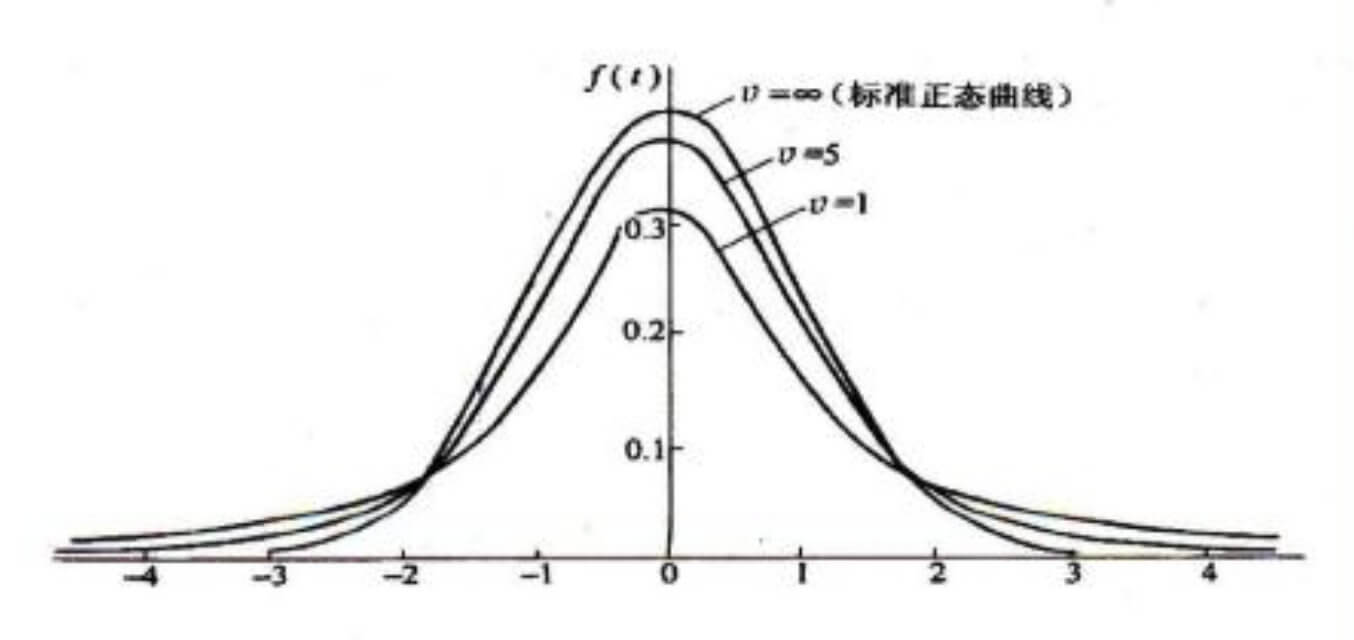t分布图有如下特征：

• 单峰分布，以0为中心，左右对称；
• t分布的曲线形态取决于自由度$v$的大小，自由度$v$越小，则t值越分散，曲线的峰部越矮而尾部翘得越高；
• 当$v$逼近$\infty$，$S_{\bar X}$逼近$\sigma_{\bar x}$，t分布逼近标准正态分布。当自由度趋于$\infty$时，t分布就是标准正态分布，故标准正态分布就是t分布的特例。

## 3.1.单样本t检验

$t=\frac{\bar X-\mu}{S_{\bar X}}=\frac{\bar X-\mu}{S/\sqrt n}=\frac{\bar X-\mu_0}{S/\sqrt n},v=n-1$

## 3.2.配对样本t检验

$t=\frac{\bar d-\mu_d}{S_{\bar d}}=\frac{\bar d-0}{S_d/\sqrt n}=\frac{\bar d}{S_d/\sqrt n},v=n-1$

### 3.2.1.配对样本t检验的应用场景举例

⚠️但是欲进行有效的假设检验，一个重要前提是测试错误率均为泛化错误率的独立采样。⚠️

## 3.3.两样本t检验

### 3.3.1.总体方差相等的t检验

$t=\frac{\bar{X_1}-\bar{X_2}}{\sqrt{\frac{(n_1-1)S_1^2+(n_2-1)S_2^2}{n_1+n_2-2}(\frac{1}{n_1}+\frac{1}{n_2})}},v=n_1+n_2-2$

1. Cochran&Cox法
2. Satterthwaite法
3. Welch法

# 4.参数检验之F检验

1 0.82 0.65 0.51
2 0.73 0.54 0.23
3 0.43 0.34 0.28
4 0.41 0.21 0.31
5 0.68 0.43 0.24

## 4.3.多个样本均数间的多重比较

### 4.3.3.SNK-q检验

SNK（Student-Newman-Keuls）检验，亦称$q$检验，适用于多个样本均数两两之间的全面比较。

# 5.非参数检验之$\chi^2$检验

$\chi^2$检验常用于推断两个总体率或构成比之间有无差异、多个总体率或构成比之间有无差别、多个样本率间的多重比较、两个分类变量之间有无关联性、多维列联表的分析和频数分布拟合优度。

👉$\chi^2$分布：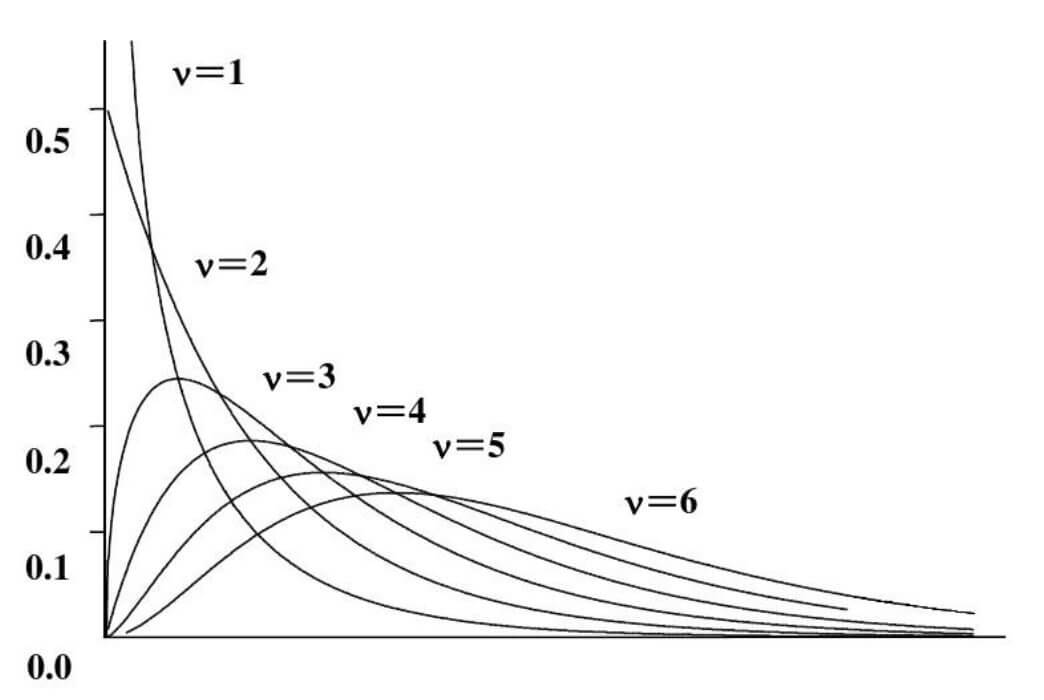$\chi^2$分布是一种连续型分布：$\chi^2$分布只有一个参数，即自由度$v$。按$\chi^2$分布的密度函数$f(\chi^2)$可给出自由度$v=1,2,3,…$的一簇$\chi^2$分布曲线（见上图）。由$\chi^2$分布曲线可见，$\chi^2$分布的形状依赖于自由度$v$的大小：

1. 当自由度$v\leqslant2$时，曲线呈$L$形；
2. 随着$v$的增加，曲线逐渐趋于对称；
3. 当自由度$v\to \infty$时，$\chi^2$分布趋近正态分布。

## 5.1.四格表资料的$\chi^2$检验

$a$ $b$ $a+b$
$c$ $d$ $c+d$

$\chi^2=\sum\frac{(A-T)^2}{T},v=(行数-1)(列数-1)$
• A为实际频数
• T为理论频数

$T_{RC}=\frac{n_R\times n_C}{n}$
• $T_{RC}$为第$R$行$C$列的理论频数
• $n_R$为相应的行合计
• $n_C$为相应的列合计

$\chi^2=\frac{(ad-bc)^2n}{(a+b)(a+c)(b+d)(c+d)},v=(行数-1)(列数-1)$

### 5.1.1.四格表资料$\chi^2$检验的校正公式

1. 当$n\geqslant 40$且所有的$T\geqslant 5$时，用$\chi^2$检验的基本公式或四格表资料$\chi^2$检验的专用公式；当$P\approx \alpha$时，改用四格表资料的Fisher确切概率法。

2. 当$n\geqslant 40$但有$1\leqslant n\lt 5$时，用四格表资料$\chi^2$检验的校正公式：$\chi^2=\sum\frac{(\mid A-T\mid -0.5)^2}{T}$或$\chi^2=\frac{(\mid ad-bc\mid-\frac{n}{2})^2n}{(a+b)(a+c)(b+d)(c+d)}$或改用四格表资料的Fisher确切概率法。

3. 当$n\lt 40$，或$T\lt 1$时，用四格表资料的Fisher确切概率法：$P=\frac{(a+b)!(c+d)!(a+c)!(b+d)!}{a!b!c!d!n!}$直接得到概率值。

## 5.2.配对四格表资料的$\chi^2$检验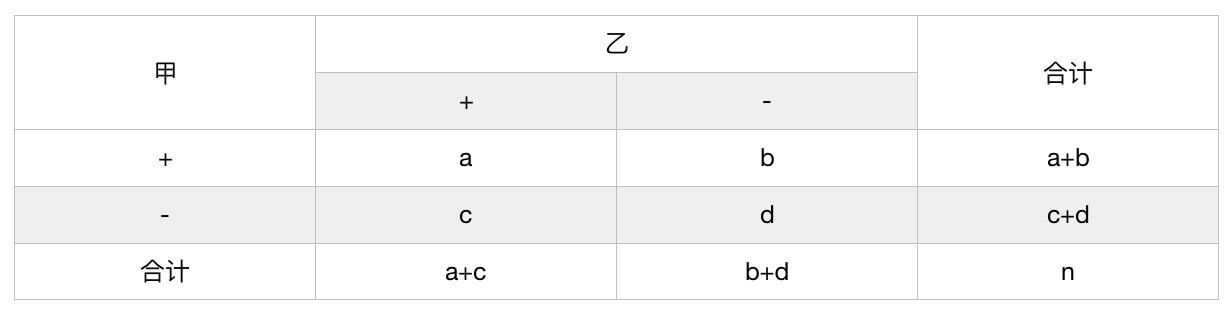• 当$(b+c)\geqslant 40$时：$\chi^2=\frac{(b-c)^2}{b+c},v=1$
• 当$(b+c)\lt 40$时，使用校正公式：$\chi^2_c=\frac{(\mid b-c \mid-1)^2}{b+c},v=1$

⚠️卡方检验中四格表和配对四格表的区别：如果是同一组人群，那么就是配对四格表；两组人群互相独立，就是四格表。

## 5.3.行$\times$列表资料的$\chi^2$检验

• 多个样本率的比较
• 两个或多个构成比的比较
• 双向无序分类资料的关联性检验

1. 多个样本率比较时，有R行2列，称为$R\times 2$表
2. 两个样本的构成比比较时，有2行C列，称$2\times C$表
3. 多个样本的构成比比较以及双向无序分类资料关联性检验时，有R行C列，称为$R\times C$表

$\chi^2=n(\sum\frac{A^2}{n_Rn_C}-1),v=(行数-1)(列数-1)$

## 5.4.多个样本率间的多重比较

### 5.4.2.实验组与同一个对照组的比较

$k$为样本率的个数。

# 6.秩转换的非参数检验

## 6.1.配对样本比较的Wilcoxon符号检验

Wilcoxon符号秩检验（Wilcoxon signed-rank test），亦称符号秩和检验。有以下两种用法：

### 6.1.1.配对样本差值的中位数和0比较

(1)

(2)

(3)

(4)=(3)-(2)

(5)

(6)
1 60 76 16 8
2 142 152 10 5
3 195 243 48 11
4 80 82 2 1.5
5 242 240 -2   1.5
6 220 220 0
7 190 205 15 7
8 25 38 13 6
9 198 243 45 9
10 38 44 6 4
11 236 190 -46   10
12 95 100 5 3

$H_0$：差值的总体中位数$=0$
$H_1$：差值的总体中位数$\neq 0$

1. 省略所有差值为0的对子数，令余下的有效对子数为n，上题中$n=11$；
2. 按11个差值的绝对值从小到大编正秩和负秩，遇差值的绝对值相等者取平均秩，称为相同秩（样本较小时，如果相同秩较多，检验结果会存在偏性，因此应提高测量精度，尽量避免出现较多的相同秩）；
3. 任取正秩和或负秩和为$T$，上题中$T=11.5$。

$u=\frac{T-n(n+1)/4}{\sqrt{\frac{n(n+1)(2n+1)}{24}-\frac{\sum (t^3_j-t_j)}{48}}}$

### 6.1.2.单个样本中位数和总体中位数比较

(1)
(1)-45.30
(2)

(3)

(4)
44.21 -1.09   1.5
45.30 0
46.39 1.09 1.5
49.47 4.17 3
51.05 5.75 4
53.16 7.86 5
53.26 7.96 6
54.37 9.07 7
57.16 11.86 8
67.37 22.07 9
71.05 25.75 10
87.37 42.07 11

## 6.2.两个独立样本比较的Wilcoxon秩和检验

1. 把两样本数据混合从小到大编秩，遇数据相等者取平均秩；
2. 以样本例数小者为$n_1$，其秩和($T_1$)为$T$，若两样本例数相等，可任取一样本的秩和($T_1$或$T_2$)为$T$。
• 当$n_1\leqslant 10$和$n_2-n_1\leqslant 10$时，查$T$界值表。
• 若$n_1\gt 10$或$n_2-n_1\gt 10$时，可用正态近似法作$u$检验。

## 6.3.完全随机设计多个样本比较的Kruskal-Wallis H检验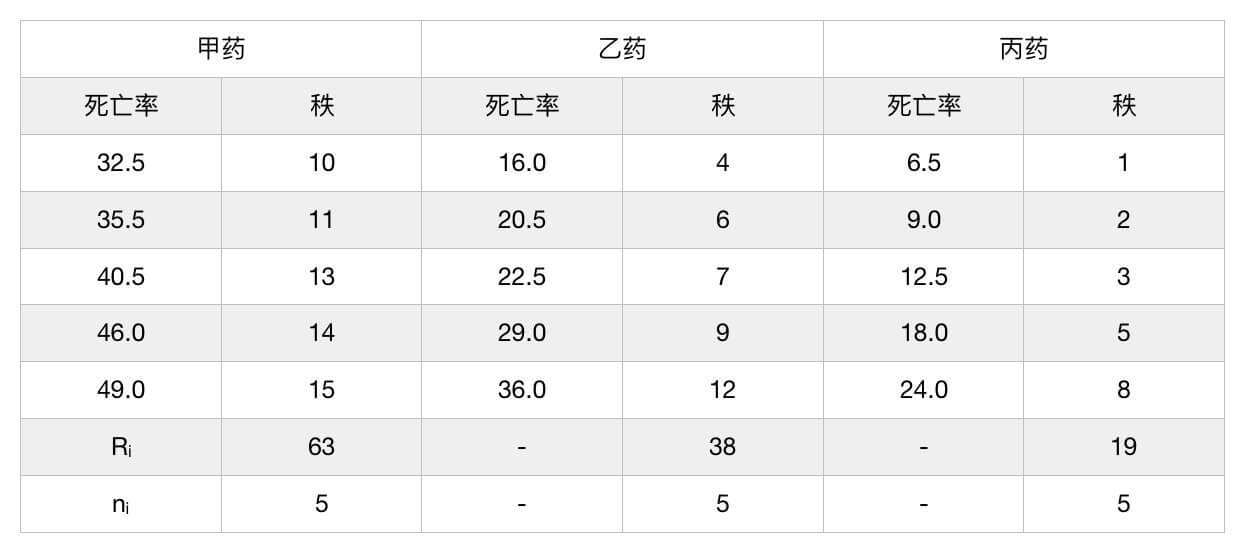1. 把3个样本数据混合从小到大编秩，遇数据相等者取平均秩；
2. 设各样本例数为$n_i(\sum n_i=N)$、秩和为$R_i$，按下式求$H$值：$H=\frac{12}{N(N+1)}(\sum \frac{R_i^2}{n_i})-3(N-1)$
3. 当各样本数据存在相同秩时，按上式算得的$H$值偏小，按下式求校正$H_C$值：$H_C=H/C,C=1-\sum(t_j^3-t_j)/(N^3-N)$
4. 查表。

## 6.4.随机区组设计多个样本比较的Friedman M检验

Friedman M检验，用于推断随机区组设计的多个相关样本所来自的多个总体分布是否有差别。

8名受试对象在相同实验条件下分别接受4种不同频率声音的刺激，他们的反应率(%)资料见下表。问4种频率声音刺激的反应率是否有差别？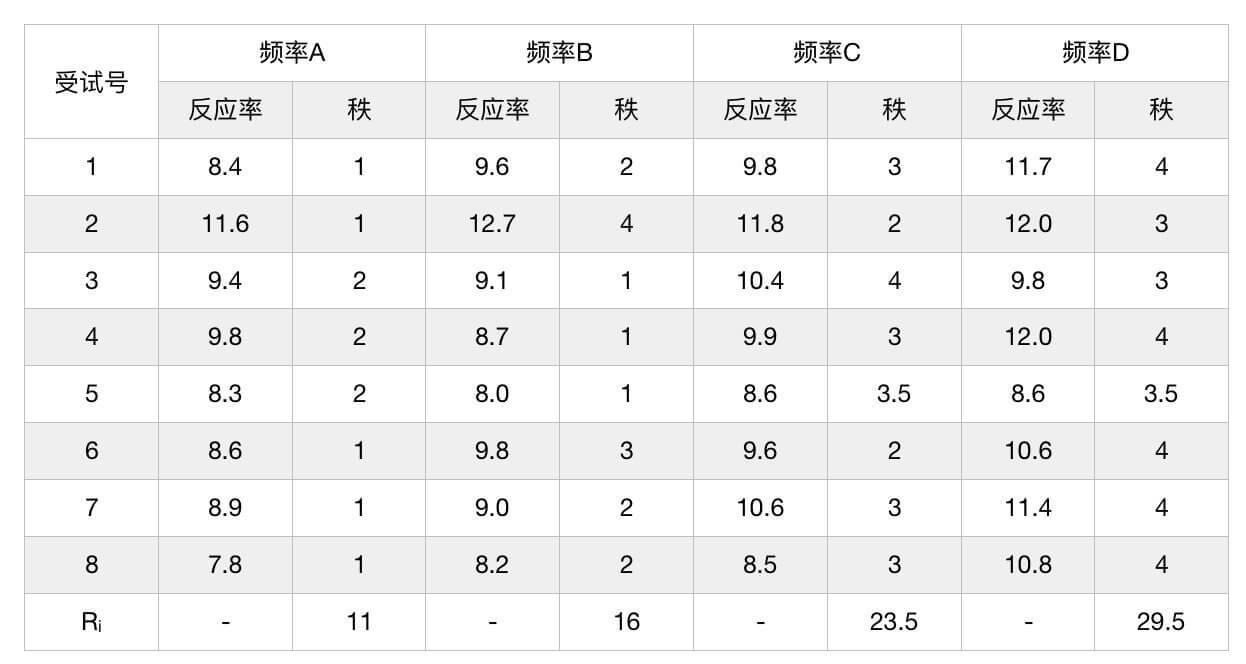• 区组个数：$n=8$
• 研究因素的水平个数：$g=4$
• 总例数：$N=ng=32$

1. 将每个区组的数据由小到大分别编秩，遇数据相等者取平均秩；
2. 计算各样本的秩和$R_i$，平均秩和为$\bar R=n(g+1)/2$；
3. 按下式求M值。
$M=\sum(R_i-\bar R)^2=\sum R_i^2-n^2g(g+1)^2/4$

$\chi^2=\frac{12M}{ng(g+1)C},C=1-\frac{\sum(t_j^3-t_j)}{n(g^3-g)},v=g-1$

👉在机器学习中的应用场景举例：

$D_1$ 1 2 3
$D_2$ 1 2.5 2.5
$D_3$ 1 2 3
$D_4$ 1 2 3

# 7.参考资料

1. 《医学统计学（第4版）》（人民卫生出版社，主编：孙振球，徐勇勇）